## Loop StatementsS2C Home « Loop Statements

In this lesson we look at the loop statements available in Java. Loop statements allow us to iterate over some code multiple times. There are two loop statements in Java, the `for` construct and the `while` construct.

### The `for` Statement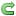Top

The `for` statement will loop through a section of code a set number of times. The `for` statement is very versatile and has two different variations known commonly as the for loop and the enhanced for loop.

The for loop contains three parts. In the first part we initialize a counter variable with a value, this only happens on initial entry to the for loop. The second part is a condition which tests the variable value at the start of each loop and if the condition is no longer `true` the for loop is exited. The final part of the `for` statement is an expression to be evaluated at the end of each iteration of the loop. This normally takes the form of a counter that is decremented or incremented.

The enchanced for loop was introduced in Java5 and implements a for-each style loop that iterates through a collection of objects in a sequential order from start to finish.

The following table shows the different forms of the `for` construct that can be used. We are using blocks of code to wrap statements which is optional when using a single statement, but good practice and will be used here.

Construct Description
`for` loop
`for ([initialization]; [condition]; [iteration]) {     statement body;}`The `initialization`, `condition`, `iteration` and `statement body` components of a `for` statement are all optional.

The following will create an infinite `for` loop :
`for (;;) {}` // Infinite loop

The following will create a `for` loop with no body:
`for (int i=1; i<5; i++);` // No body

The `initialization` component is generally an assignment statement that sets the initial value of a control variable used to control iteration of the loop.

The `condition` component is a conditional expression that is always tested againt the control variable for `true` before each iteration of the loop. So if this is `false` to begin with then any `statement body` component will never be executed.

The `iteration` component is an expression that determines the amount the control variable is changed for each loop iteration.

The `statement body` is executed each time the `condition` component returns `true` when tested against the control variable.
`enhanced for` loop
`for (declaration : expression) {     statement body}`The `declaration` component declares a variable of a type compatible with the collection to be accessed which will hold a value the same as the current element within the collection.

The `expression` component can be the result of a method call or an expression that evalutes to a collection type.

The `statement body` is executed each time an element of the collection is iterated over.

We will go through the different forms of the `for` construct one at a time, using some code to make understanding of the above table easier.

#### The `for` Construct and `break`Top

The `for` construct will loop through a section of code a set number of times dependant upon the components set for the loop and their values. Lets look at some code to illustrate how this works:

``````
/*
for Construct
*/
public class UsingFor {

public static void main (String[] args) {
for (int i=1; i<13; i++) {
System.out.println("The square of " + i + " = " + i*i);
}
for (int i=1; i<13; i++) {
// Using a break to exit loop
if (i == 6) { break; }
System.out.println("The square of " + i + " = " + i*i);
}
}
}

``````

Save, compile and run the file in directory   c:\_BeginningJava5 in the usual way.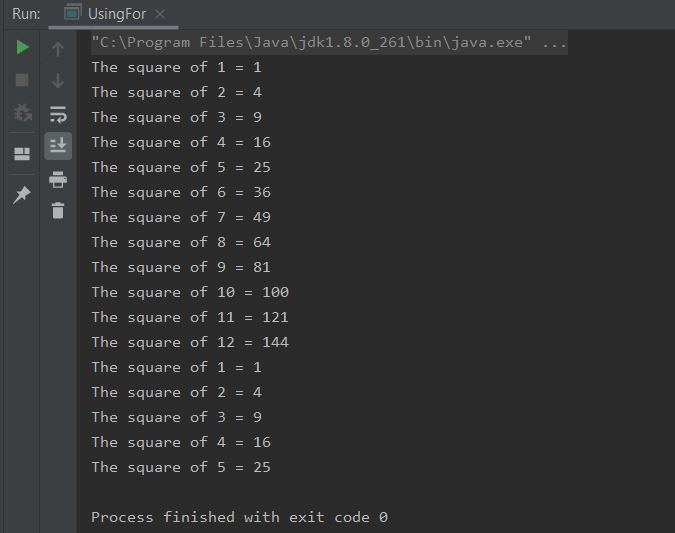In the first loop what we are doing here is using the control variable (i) and squaring it for the output while it is less than 13. After each iteration we increment the control variable by 1. In the second loop we `break` from the loop when the control variable (i) reaches 6. The `break` statement forces an exit from the loop immediately. Did you notice also that we use the variable `i` twice with no problem. This is because this variable is created and exists only as long as the scope it was created in, in this case the `for` construct. Take a look at the Method Scope lesson for a refresher on local variable scope.

#### Using A Nested `for` Construct and `break`Top

We can also nest the `for` construct within another `for` construct (or any other construct for that matter). Lets look at examples of this:

``````
/*
Nested for Construct
*/
public class NestedFor {

public static void main (String[] args) {
for (int i=1; i<11; i++) {
System.out.println("\nThe " + i + " times table: ");
for (int j=1; j<11; j++) {
System.out.print(j + "*" + i + "=" + j*i + ": ");
}
}
for (int i=1; i<11; i++) {
System.out.println("\nThe " + i + " times table: ");
for (int j=1; j<11; j++) {
// Using a break to exit inner loop
if (j == 4) { break; }
System.out.print(j + "*" + i + "=" + j*i + ": ");
}
}
}

``````

Save, compile and run the file in directory   c:\_BeginningJava5 in the usual way.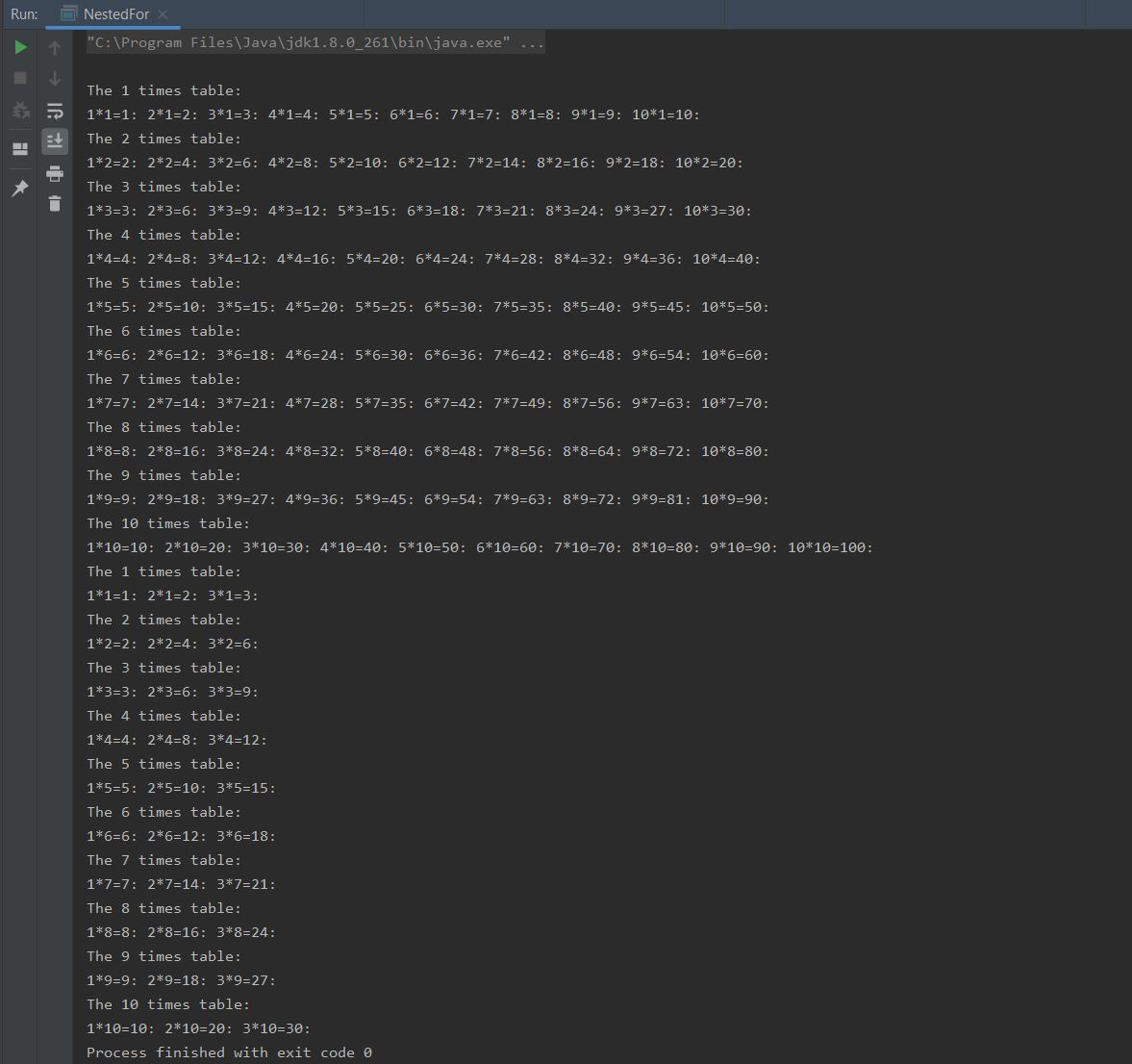What we are doing here is running a loop within a loop to print off times tables up to 10. So the inner `for` loop runs 10 times for each outer `for` loop iteration. In the second example we break from the inner loop during the fourth iteration. This is just to show that using the `break` statement forces an exit from the loop you are in not any outer loops.

#### Using the `continue` StatementTop

We can also use the `continue` statement within any loop construct. For a `for` construct, the `continue` statement will stop this iteration of the loop and continue at the start of the next iteration. Lets look at an example of this:

``````
/*
Using continue in a for Construct
*/
public class UsingForContinue {

public static void main (String[] args) {
for (int i=1; i<13; i++) {
// Using a continue to force an iteration
if ((i%2) == 0) { continue; }
System.out.println("The square of " + i + " = " + i*i);
}
}
}

``````

Save, compile and run the file in directory   c:\_BeginningJava5 in the usual way.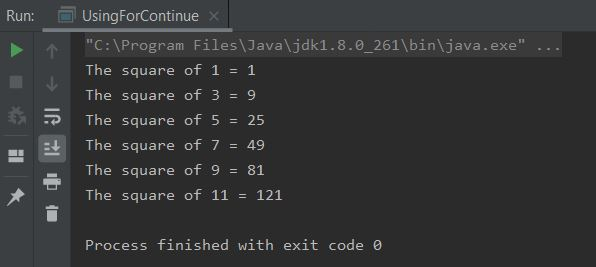In this variation we are checking to see if the control variable (i) leaves no remainder. If this is the case we are forcing a `continue` to the next iteration. For this example it means we are only printing out odd squares within the specified range.

#### Using the `break` to a labelled StatementTop

We saw how the `break` statement worked with the `for` construct in earlier examples. There is a variation where we can `break` to a labelled statement. Breaking to a labelled statement causes program execution to resume after the labelled block. Lets look at examples of this:

``````
/*
Using break to a label in a for Construct
*/
public class UsingLabelBreak {

public static void main (String[] args) {
label1:
for (int i=1; i<11; i++) {
System.out.println("\nThe " + i + " times table: ");
for (int j=1; j<11; j++) {
// Using a break to label to exit loop
if (j == 4) { break label1; }
System.out.print(j + "*" + i + "=" + j*i + ": ");
}
}
System.out.println("\n\n****");
for (int i=1; i<11; i++) {
System.out.println("\nThe " + i + " times table: ");
label2:
for (int j=1; j<11; j++) {
// Using a break to label to exit loop
if (j == 4) { break label2; }
System.out.print(j + "*" + i + "=" + j*i + ": ");
}
}
}
}

``````

Save, compile and run the file in directory   c:\_BeginningJava5 in the usual way.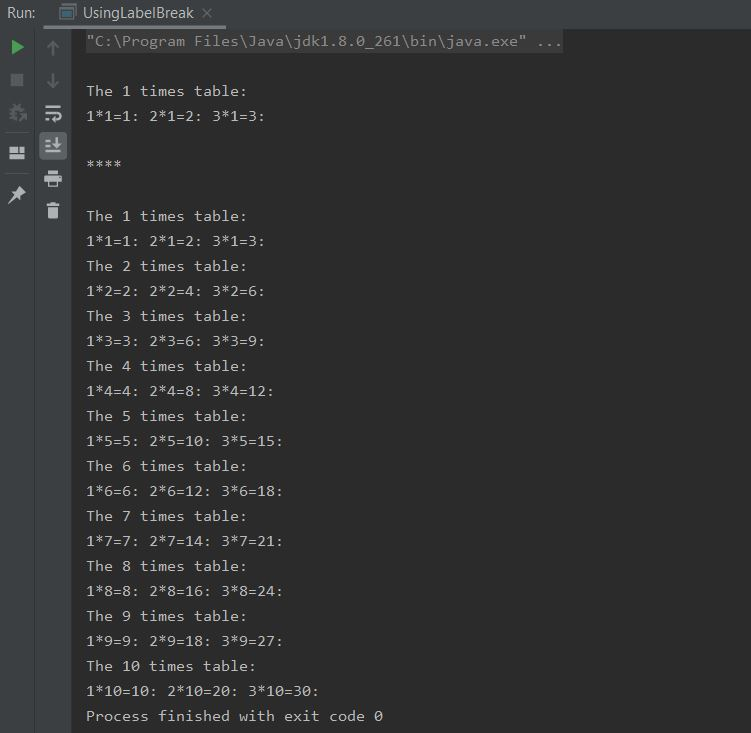In the first loop above we break to the `label1:` label, which results in processing continuing after the outer loop. So in reality we have broken out of both loops. In the second example we break to the `label2:` label, which breaks us out of the inner loop only. So processing will continue with the next iteration of the outer loop. So when using the `label` statement with the `break` statement positioning matters.

#### The `enhanced for` ConstructTop

The `enhanced for` construct will loop through each element within a collection. Lets look at some code to illustrate how this works:

``````
/*
enhanced for Construct
*/
public class EnhancedFor {

public static void main (String[] args) {
int [] anArray = {22,33,44,55};
for (int i : anArray) {
System.out.println("Array element = " + i);
}
}
}

``````

Save, compile and run the file in directory   c:\_BeginningJava5 in the usual way.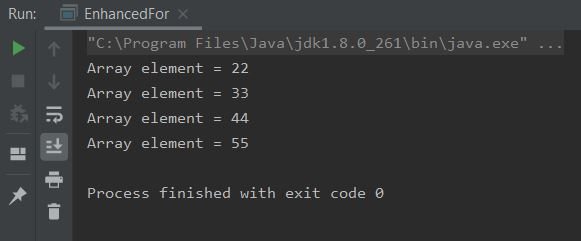What we are doing here is creating an array collection of numbers; we will expand on arrays in the Arrays lesson. We then use the `enhanced for` construct to iterate over the array collection.

### The `while` StatementTop

The `while` statement can be used to loop through a section of code while a condition remains `true`. The `while` statement has two different variations known commonly as the while loop and the do-while loop.

The following table shows the different forms of the `while` construct that can be used. We are using blocks of code to wrap statements which is optional when using a single statement, but good practice and will be used here.

Construct Description
`while` loop
`while (condition) {     statement body;}`The `condition` can be any expression that results in a boolean and the loop will continue while the expression remains `true`, processing the `statement body` on each pass.

When the `condition` returns `false` the loop ends and control is passed to the next line following the loop.

Therefore if the `condition` starts as `false` the loop will never be entered.
`do while` loop
`do {     statement body;} while (condition);`Unlike the normal `while` loop the `statement body` is processed before the `condition` is tested. The `condition` can be any expression that results in a boolean and the loop will continue while the expression remains `true`.

When the `condition` returns `false` the loop ends and control is passed to the next line following the loop.

Therefore even if the `condition` starts as `false` the loop will always execute at least once.

We will go through the different forms of the `while` construct one at a time, using some code to make understanding of the above table easier.

#### The `while` Construct and `break`Top

The `while` construct will loop through a section of code while a condition remains `true`. Lets look at some code to illustrate how this works:

``````
/*
while Construct
*/
public class UsingWhile {

public static void main (String[] args) {
int i = 1;
while (i < 13) {
System.out.println("The square of " + i + " = " + i*i);
i++;
}
i = 1;
while (i < 13) {
// Using a break to exit loop
if (i == 6) { break; }
System.out.println("The square of " + i + " = " + i*i);
i++;
}
}
}

``````

Save, compile and run the file in directory   c:\_BeginningJava5 in the usual way.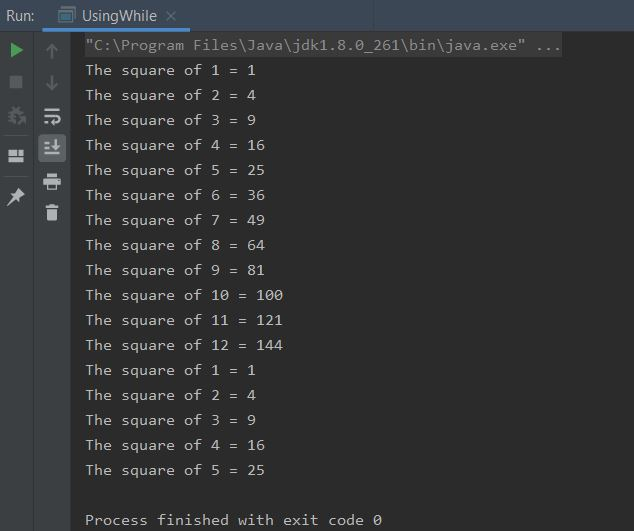In the first loop what we are doing here is using the control variable (i) and squaring it for the output while it is less than 13. We increment the control variable by 1 just before exiting the `while` loop each time. In the second loop we `break` from the loop when the control variable (i) reaches 6. The `break` statement forces an exit from the loop immediately.

#### Using a Nested `while` Construct and `break`Top

We can also nest the `while` construct within another `while` construct (or any other construct for that matter). Lets look at examples of this:

``````
/*
Nested while Construct
*/
public class NestedWhile {

public static void main (String[] args) {
int i = 1;
while (i < 11) {
System.out.println("\nThe " + i + " times table: ");
int j = 1;
while (j < 11) {
System.out.print(j + "*" + i + "=" + j*i + ": ");
j++;
}
i++;
}
i = 1;
while (i < 11) {
System.out.println("\nThe " + i + " times table: ");
j = 1;
while (j < 11) {
// Using a break to exit inner loop
if (j == 4) { break; }
System.out.print(j + "*" + i + "=" + j*i + ": ");
j++;
}
i++;
}
}
}

``````

Save, compile and run the file in directory   c:\_BeginningJava5 in the usual way.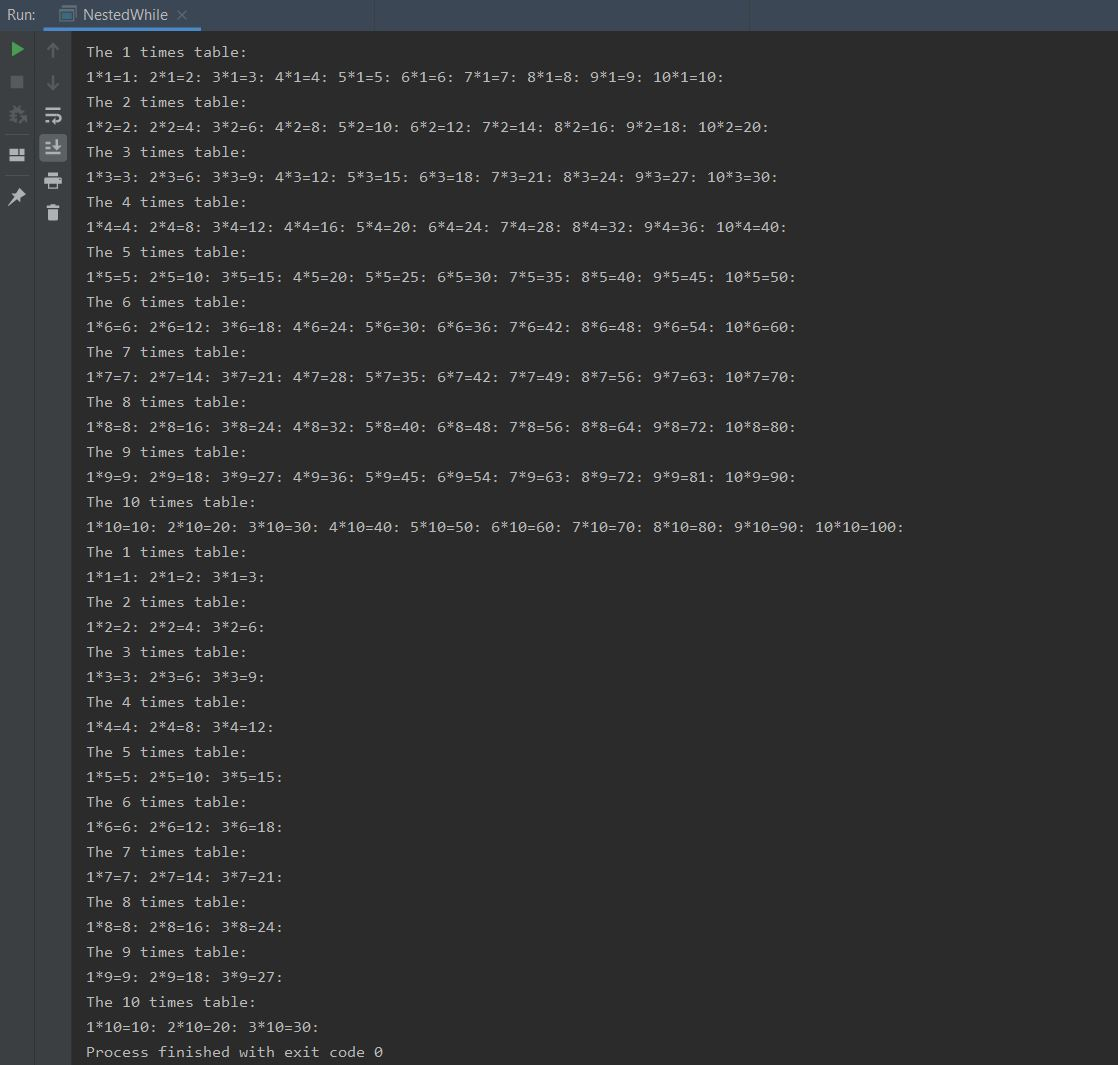What we are doing here is running a loop within a loop to print off times tables up to 10. So the inner `while` loop runs 10 times for each outer `while` loop iteration. In the second example we break from the inner loop during the fourth iteration. This is just to show that using the `break` statement forces an exit from the loop you are in not any outer loops.

#### Using the `continue` StatementTop

For a `while` construct, the `continue` statement will pass control back to the start of the loop immediately. Lets look at an example of this:

``````
/*
Using continue in a while Construct
*/
public class UsingWhileContinue {

public static void main (String[] args) {
int i = 1;
while (i < 13) {
// Using a continue to force an iteration
if ((i%2) == 0) { i++; continue; }
System.out.println("The square of " + i + " = " + i*i);
i++;
}
}
}

``````

Save, compile and run the file in directory   c:\_BeginningJava5 in the usual way.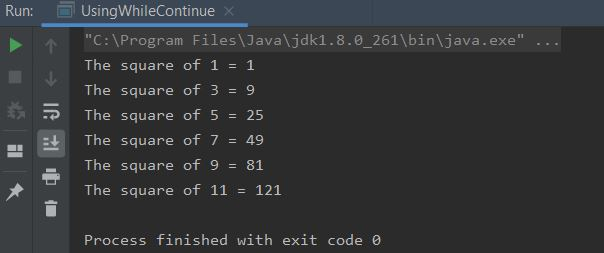In this variation we are checking to see if the control variable (i) leaves no remainder. If this is the case we are forcing a `continue` to the next iteration. For this example it means we are only printing out odd squares within the specified range. If you notice when we hit this condition we have to increment our control variable before passing control back to the top of the loop. Unlike the `for` construct, the `while` has no auto increment and hence if we do not increment, we end up in an infinite loop situation.

#### Using the `break` to a labelled StatementTop

We saw how the `break` statement worked with the `while` construct in earlier examples. There is a variation where we can `break` to a labelled statement. Breaking to a labelled statement causes program execution to resume after the labelled block. Lets look at examples of this:

``````
/*
Using break to a label in a while Construct
*/
public class UsingWhileLabelBreak {

public static void main (String[] args) {
int i = 1, j = 1;
label1:
while (i<11) {
System.out.println("\nThe " + i + " times table: ");
j = 1;
while (j<11) {
// Using a break to label, to exit outer loop
if (j == 4) { i++; break label1; }
System.out.print(j + "*" + i + "=" + j*i + ": ");
j++;
}
i++;
}
System.out.println("\n\n****");
i = 1;
while (i<11) {
System.out.println("\nThe " + i + " times table: ");
j = 1;
label2:
while (j<11) {
// Using a break to label, to exit inner loop
if (j == 4) {  j++; break label2; }
System.out.print(j + "*" + i + "=" + j*i + ": ");
j++;
}
i++;
}
}
}

``````

Save, compile and run the file in directory   c:\_BeginningJava5 in the usual way.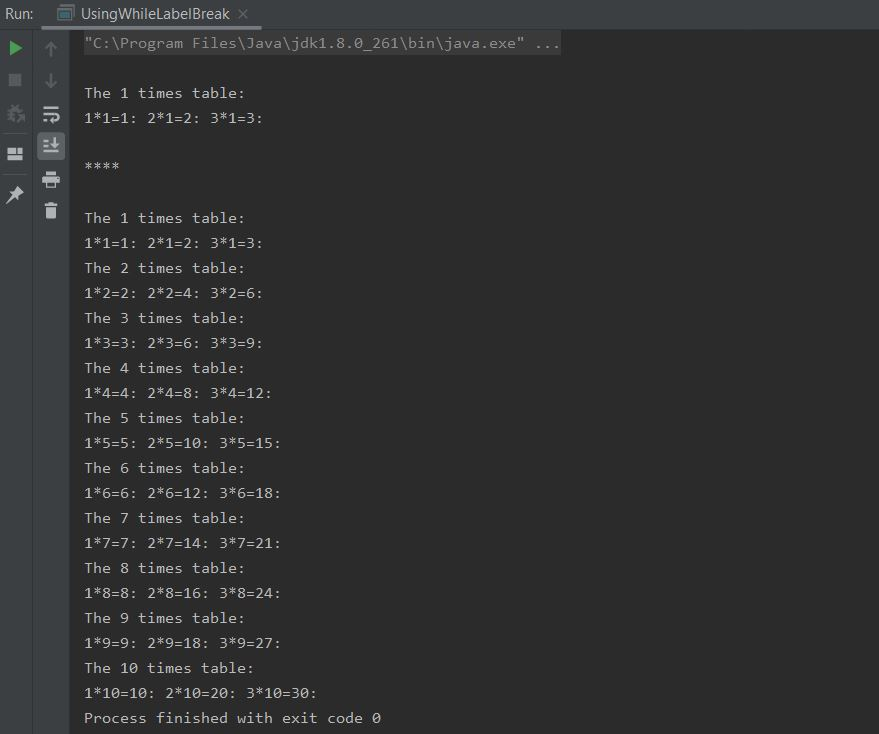In the first loop above we break to the `label1:` label, which results in processing continuing after the outer loop. So in reality we have broken out of both loops. In the second example we break to the `label2:` label, which breaks us out of the inner loop only. So processing will continue with the next iteration of the outer loop. So when using the `label` statement with the `break` statement positioning matters.

#### The `do while` ConstructTop

The `do while` construct will loop through a section of code while a condition remains `true`. Because the statement body is executed before the condition is tested the `do while` construct will always execute at least once. Lets look at some code to illustrate how this works:

``````
/*
do while Construct
*/
public class UsingDoWhile {

public static void main (String[] args) {
int i = 13;
while (i < 13) {
System.out.println(""WHILE. The square of " + i + " = " + i*i);
i++;
}
do {
System.out.println("DO WHILE. The square of " + i + " = " + i*i);
i++;
} while (i < 13);
}
}

``````

Save, compile and run the file in directory   c:\_BeginningJava5 in the usual way.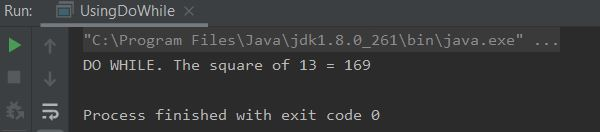In the `while` loop the control expression returns `false` and so the loop never runs. In the `do while` loop the control expression still returns `false` but the loop is always guaranteed to execute at least once, which is what happens in this case.

Apart from this the `do while` behaves exactly the same as a normal `while` loop and can use the `break`, `continue` and `label` statements in the same way.

## Lesson 8 Complete

In this lesson we investigated the loop statements available for use in Java5.

### What's Next?

We begin a new section about objects and classes and start the section with a look at arrays.